Kotlin: An Illustrated Guide • Chapter 1

# Variables, Expressions, and Types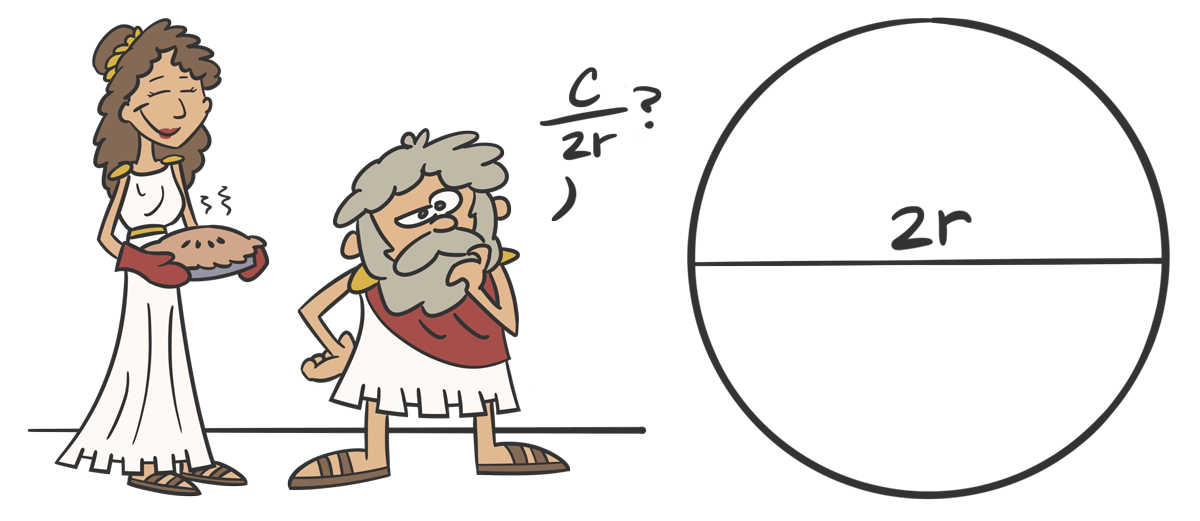Archimedes is deciding what to name his discovery...

## So you want to be a Kotlin programmer?

You’ve come to the right place!

This series will take you through the fundamentals of Kotlin, gently introducing you to the core concepts of the language, in order to help you become a proficient Kotlin developer. Even if you’re a seasoned pro, it’s important to know the fundamentals in order to establish a solid foundation of understanding, so that you can be as effective as possible.

Your adventure starts here in Chapter 1, where we’ll cover the basics of variables, expressions, and types.

Let’s get to it!

## Introduction to Variables

This is a circle.Thousands of years ago, a Greek fellow named Archimedes roughly figured out how to measure the length of the outside of a circle, called the circumference. You probably remember the equation the good old days of junior high school:“The circumference of a circle is equal to 2 times π times the radius of the circle”.

In the equation above, the letter `r` isn’t a number itself. It’s a letter that represents the radius - a number that measures the distance between the center of the circle and the edge of the circle.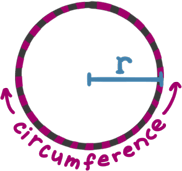We call `r` a variable, because the radius can vary, depending on the size of the circle. In other words, the variable `r` isn’t a number itself; it’s just sort of a “bucket” that holds a number - any number.Variables aren’t just for algebra and geometry. They’re also used all the time in programming, where they serve the same purpose: holding values. In Kotlin, you can create a variable and put a number into it like this:

``var r = 5.2``

This code is actually doing two things on one line.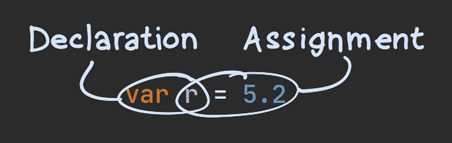1. When we write `var r`, we’re declaring a new variable called `r`. You can think of declaring a variable as creating a bucket.
2. When we write `r = 5.2`, we’re assigning the value of `5.2` to the variable `r`. In other words, we’re putting the number `5.2` into the bucket.Let’s break down the three main parts of this line of code: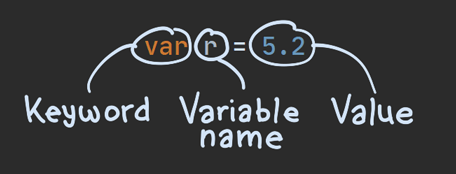In this code…

• `var` is a keyword that tells Kotlin to create a new variable.
• `r` is the name of the variable. You might also hear this referred to as the variable’s identifier.
• The number `5.2` is the value that is being assigned.

`var` is just one of many keywords that we’ll see as we continue to learn Kotlin. The important thing to remember about keywords is that they can’t be used as the name of your own things. For example, you can’t use `var` as the name of the variable:

``var var = 5``
Error

We’ll come across other keywords in future chapters.

### Reassigning Variables

As we noted above, in the equation 2πr, the variable `r` can represent different numbers in different situations.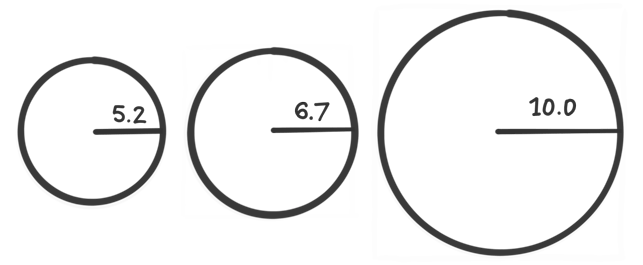In Kotlin, if you decide that you want `r` to represent a different number, you can just reassign it, as shown in the second line here:

``````var r = 5.2
r = 6.7``````

When reassigning this variable, we didn’t need to use the `var` keyword again, because the variable was already declared on that first line.

### Variables that Cannot Be Reassigned

Let’s look at that circumference equation again:The Greek letter `π` (pronounced like the word “pie”) is very different from the variable `r`. Whereas `r` can hold any number in it, `π` only ever holds one very specific number, which we’ll abbreviate to 3.14.

In the same way, when programming in Kotlin, there are times when you want to make sure that a variable never changes.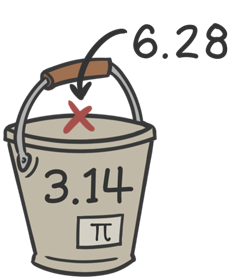In those cases, instead of declaring it with `var`, you declare it with `val`, like this:

``val π = 3.14``

Now, if you try to reassign `π`, you’ll get an error:

``````val π = 3.14
π = 1.23``````
Error

Once you put something in this bucket, you can never replace it!

Declaring your variables with `val` is a great way to make sure that you don’t accidentally change something that shouldn’t change. In fact, it’s a great idea to always start with `val`, and only switch to `var` when you absolutely need to.

### Naming Variables

It’s been fun using the letter `π` in our code, but unless you live in Greece, you probably don’t have it on your keyboard. From here on out, we’ll make life easier for everyone by naming it `pi` instead. Also, instead of `r`, we’ll name it `radius`, so that any other developers who come along later will know exactly what that variable represents - we don’t want others to have to guess what the letter `r` stands for!

Sometimes you need more than one word for a variable’s identifier. In Kotlin, it’s customary to start the first word lowercased, and then capitalize the remaining words, like this:

``var radiusOfTheCircle = 5.2``

Now that you’ve got down the basics for declaring and assigning variables, we can start assigning more than just simple numbers - we can start assigning more complex calculations, such as our circumference equation! Let’s dive into expressions!

## Expressions and Statements in Kotlin

Let’s look at that equation again:We’ve already created a variable for `pi` and a variable for `radius`, so now we just need Kotlin to do some math for us, and we can get the circumference of any circle, regardless of how big that circle is.

All we have to do is multiply together `2`, `pi`, and `radius`. In Kotlin, as in most programming languages, multiplication isn’t represented with an `x`, it’s represented with an asterisk: `*`, so our code can look like this:

``````var radius = 5.2
val pi = 3.14

val circumference = 2 * pi * radius``````

So far we’ve only assigned simple values - such as `5.2` and `3.14`. This is the first time we’re assigning something more complex: `2 * pi * radius`.

When Kotlin sees this, it simply calculates the result for you - it multiplies `2` times `pi` times `radius`, and then, of course, it takes the resulting value and assigns it to the variable named `circumference`. In this case (with a radius of `5.2`), `circumference` will equal `32.656`.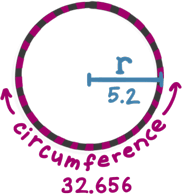Since `2 * pi * radius` can be calculated into a value like this, we say that it can be evaluated. Code that can be evaluated is called an expression. Here are a few examples of expressions:

• `2 + 3`
• `2 * pi * r`
• `pi * r * r`

Variables by themselves are also expressions - they evaluate to whatever value they hold:

• `radius`
• `pi`

When you type out a number by hand (as opposed to typing a variable), it’s called a number literal. Literals themselves are also expressions - they evaluate to themselves! Here are a few examples:

• `2`
• `5.2`
• `3.14`

All of those examples will evaluate to some value. On the other hand, when you have a chunk of code that does not evaluate to a value, it’s called a statement.

Here’s an easy rule of thumb to know if you’ve got an expression or a statement:

Rule: If you can assign a chunk of code to a variable, it’s an expression. Otherwise, it’s a statement.

Let’s apply this rule to the first expression from each of the three lists above (`2 + 3`, `radius`, and `2`). The parts highlighted below are the expressions:

``````val a = 2 + 3
val c = 2``````

Since each of those can be assigned to a variable, they’re all expressions.

The only statement we’ve seen so far is the assignment statement, such as `val pi = 3.14`. Applying our rule of thumb to an assignment statement looks confusing, of course, because we’re using an assignment on an assignment (yikes!):

``val a = val pi = 3.14``
Error

If you try to do this, Kotlin gives you a helpful error message, “Expecting an expression”. If you ever see this error message, it just means you tried to use a statement where Kotlin wanted an expression.

The distinction between statements and expressions is important as you’re learning Kotlin, and we’ll use those terms often in this series.

So far, whether we’ve used literals or complex expressions, we’ve still only ever assigned numbers to variables. But there are lots of different things that variables can hold! Let’s explore some of these things next.

## Types: Different Kinds of Values

In Kotlin, different variables can hold different kinds of values. The kind of value that a variable holds is called its type.

Let’s take another look at the variables `radius` and `pi`:

``````var radius = 5.2
val pi = 3.14``````

In this case, both `radius` and `pi` represent numbers that have a decimal point, which is a type that Kotlin calls a `Double`. If you want to, you can tell Kotlin the type of the variable yourself, like this:

``````var radius: Double = 5.2
val pi: Double = 3.14``````

When we do this, we are explicitly specifying the type.

Very often, you do not have to specify the type of a variable yourself. In that case, Kotlin will do its best to infer the type based on whatever it is that you’re assigning to the variable. That process is called type inference.

So, when we write this…

``var radius = 5.2``

…then Kotlin can tell that `5.2` is a `Double`, so it automatically uses `Double` as the type of `radius`.

In addition to `Double`, there are some other basic types that are good to know about! Let’s take a look at some of those now.

### Integers

So far we’ve only used numbers that have a decimal point in them, such as `5.2` and `3.14`. But you might also use a number that does not have a decimal point, such as just `5` or `3`.

These kinds of numbers are called integers, and in Kotlin the type for an integer is just called `Int` for short. Here’s an example of creating an integer variable:

``val numberOfDogs: Int = 3``

### Booleans

Sometimes you want a variable to hold a value that is either on or off, yes or no, true or false, and so on.

In those cases, you want a `Boolean` variable.

``````val earthIsRound: Boolean = true
val earthIsFlat: Boolean = false``````

Why is it called a `Boolean`?

It’s named after a British chap from the 1800s, George Boole, who created a branch of algebra that works with true and false values instead of numbers.

Awesome.

### Strings

You can also store text into a variable. The fancy programmer word for this is string, because it’s a bunch of characters - such as letters, numbers, and symbols - “strung” together:In Kotlin, the type for this is called `String`, and you can create a `String` variable like this:

``val text: String = "This is a string"``

### Other Types

These are just some common types of variables. As we continue this series, we’ll find out how we can create our own types - classes - which build upon these basic types that we just looked at.

### Types and Reassignment

In Kotlin, the type of each variable is established when you’re writing the code, and its type will never change (unless you rewrite the code). This is why we call it static typing. Once a variable has been declared with a particular type, no other type of value can go into it. For example, if we create a variable of type `Int`, we can’t later reassign it with a `String`:

``````var numberOfDogs: Int = 5
numberOfDogs = "five"``````
Error

In future chapters, we’ll see how this static typing plays out in more ways than just reassigning variables. We’ll also see how some types can have subtypes.

But we’re getting ahead of ourselves - for now, let’s wrap up this chapter!

## Summary

In this chapter, we looked at:

• Variables
• Using `var` for variables that can be reassigned.
• Using `val` for variables that are read-only.
• Expressions and Statements
• Expressions can be evaluated to a value.
• Statements do not evaluate to a value.
• You can try assigning a chunk of code to a variable to see if it’s a statement or an expression.
• Basic Types
• Number types like `Double` and `Int`.
• The `Boolean` type for `true` and `false` values.
• The `String` type for text values.

Now that we have a good grasp on variables, it’s time to put them together in exciting new ways! Stay tuned for chapter 2, where we’ll learn all about functions in Kotlin!

Thanks to Matt McKenna, Jacob Rakidzich, and Doug Smith for reviewing this article.